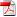### Pavol Zajac, and Peter Spacek

#### A New Type of Signature Scheme Derived from a MRHS Representation of a Symmetric Cipher

We propose a new concept of (post-quantum) digital signature algorithm derived from a symmetric cipher. Key derivation is based on a system of Multiple-Right-Hand-Sides equations. The source of the equations is the encryption algorithm. Our trapdoor is based on the difficulty of creating a valid transcript of the encryption algorithm for a given plaintext (derived from the signed message): the signer can use the encryption algorithm, because he knows the secret key, and the verifier can only check that the solution of the equation system is correct. To further facilitate the verification, we use techniques from coding theory. Security of the system is based on the difficulty of solving MRHS equations, or equivalently on the difficulty of the decoding problem (both are NP hard).

Reference:
DOI: 10.36244/ICJ.2019.4.4

DownloadPlease cite this paper the following way:

Pavol Zajac and Peter Špaček, "A New Type of Signature Scheme Derived from a MRHS Representation of a Symmetric Cipher", Infocommunications Journal, Vol. XI, No 4, December 2019, pp. 23-30. DOI: 10.36244/ICJ.2019.4.4